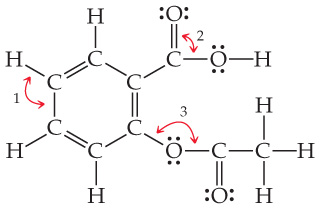Chemistry Practice Problems Bond Angles Practice Problems Solution: The compound with the following Lewis structure is...

⚠️Our tutors found the solution shown to be helpful for the problem you're searching for. We don't have the exact solution yet.

# Solution: The compound with the following Lewis structure is acetylsalicylic acid, better known as aspirin: .What are the approximate values of the bond angles labeled 1, 2, and 3?

###### Problem

The compound with the following Lewis structure is acetylsalicylic acid, better known as aspirin:.

What are the approximate values of the bond angles labeled 1, 2, and 3?

Bond Angles

Bond Angles

#### Q. Consider the Lewis structure for glycine, the simplest amino acid: What are the approximate bond angles at the nitrogen?

Solved • Mon Nov 12 2018 15:48:57 GMT-0500 (EST)

Bond Angles

#### Q. In a trigonal-bipyramidal geometry, there are two types of positions for the outer atoms.What is the bond angle formed by an axial atom, the central a...

Solved • Mon Nov 12 2018 15:48:55 GMT-0500 (EST)

Bond Angles

#### Q. Use VSEPR to predict the geometry (including bond angles) about each interior atom of methyl azide (CH3N3), and make a sketch of the molecule. Would y...

Solved • Mon Nov 12 2018 12:41:36 GMT-0500 (EST)

Bond Angles

#### Q. Suppose that a molecule has four bonding groups and one lone pair on the central atom. Suppose further that the molecule is confined to two dimensions...

Solved • Mon Nov 12 2018 12:41:11 GMT-0500 (EST)